• 2021-08-27 16:35:28

## 1.和的界限

	 - 数学归纳法
- 放缩法
- 分裂法
- 积分法


## 2.常系数线性递归方程

称递归方程为k次常系数线性递归方程，若f(n)=0，则称方程是齐次的，否则称之为非齐次的
T ( n ) = a 1 T ( n − 1 ) + a 2 T ( n − 2 ) + a 3 T ( n − 3 ) + ⋯ + a k T ( n − k ) + f ( n ) . T(n) =a_1T(n-1)+a_2T(n-2)+a_3T(n-3)+\dots+a_kT(n-k)+f(n).
称一元k次方程为递归方程的特征方程
x k − ( a 1 x k − 1 + a 2 x k − 2 + a 3 x k − 3 + ⋯ + a k ) = 0. x^k-(a_1x^{k-1}+a_2x^{k-2}+a_3x^{k-3}+\dots+a_k)=0.

定理1
设特征方程的根是 r 1 , r 2 , . . . , r s r_1,r_2,...,r_s ，每个根的重数依次为 m 1 , m 2 , . . . , m s m_1,m_2,...,m_s ,则常系数齐次线性递归方程的通解是 T ( n ) = ∑ i = 1 s r i n ( c i 1 + c i 2 × n + ⋯ + c i m i × n m i − 1 ) T(n)=\sum_{i=1}^sr_i^n(c_{i1}+c_{i2}\times n+\dots+c_{im_i}\times n^{m_i-1}) 其中系数 c i 1 ， c i 2 ， … c_{i1}，c_{i2}，\dots 由方程的初始条件 T ( 0 ) , T ( 1 ) , T ( k − 1 ) T(0),T(1),T(k-1) 唯一确定
定理2
如果非齐次递归方程中 f ( n ) = ∑ i = 1 d e i n i f(n)=\sum_{i=1}^de_in^i ，1是特征方程的m重根（若1不是根则m=0），则方程的一个特解为 T p ( n ) = p 0 + p 1 n + ⋯ + p d + m n d + m T_p(n)=p_0+p_1n+\dots+p_{d+m}n^{d+m} ，待定系数法，将其代入递归方程解出系数，方程的解为 T ( n ) = T h ( n ) + T p ( n ) T(n)=T_h(n)+T_p(n) 其中 T h ( n ) T_h(n) 是相应齐次方程的通解
定理3
如果非齐次递归方程中 f ( n ) = c a n f(n)=ca_n ，且a是特征方程的m重根，则方程的一个特解为 T p ( n ) = ( p 0 + p 1 n + ⋯ + p m n m ) a n T_p(n)=(p_0+p_1n+\dots+p_{m}n^{m})a^n ，待定系数法，将其代入递归方程解出系数，方程的解为 T ( n ) = T h ( n ) + T p ( n ) T(n)=T_h(n)+T_p(n) 其中 T h ( n ) T_h(n) 是相应齐次方程的通解

## 3.非常系数线性递归方程

通过两边同乘，错位相减，两边同除等技巧将方程转换成常系数方程
求解形如 T ( n ) = g ( n ) T ( n − 1 ) + f ( n ) T(n)=g(n)T(n-1)+f(n) 的方程时，可令 T ( n ) = g ( n ) g ( n − 1 ) … g ( 1 ) S ( n ) T(n)=g(n)g(n-1)\dots g(1)S(n) ，代入方程得 g ( n ) g ( n − 1 ) … g ( 1 ) S ( n ) = g ( n ) g ( n − 1 ) … g ( 1 ) S ( n − 1 ) + f ( n ) g(n)g(n-1)\dots g(1)S(n)=g(n)g(n-1)\dots g(1)S(n-1)+f(n) ，于是 S ( n ) = S ( n − 1 ) + f ( n ) / [ g ( n ) g ( n − 1 ) … g ( 1 ) ] S(n)=S(n-1)+f(n)/[g(n)g(n-1)\dots g(1)] ，用迭代法可解得 S ( n ) = S ( 0 ) + ∑ i = 1 n f i / [ g ( i ) g ( i − 1 ) … g ( 1 ) ] S(n)=S(0)+\sum_{i=1}^nf_i/[g(i)g(i-1)\dots g(1)] ，进而可解出方程

## 4.生成函数法求解复杂递归方程

构造递归方程的生成函数为形式级数 G ( z ) = T ( 1 ) z + T ( 2 ) z 2 + ⋯ + T ( n ) z n + … G(z)=T(1)z+T(2)z^2+\dots+T(n)z^n+\dots 根据递归方程的形式，得到生成函数满足的方程，解出生成函数表达式，再将其写成级数的形式（泰勒展开）， z n z^n 的系数即为 T ( n ) T(n)

## 5.分治算法递归方程

分治算法中时间复杂度的表达式往往形如 { T ( n ) = a T ( n / b ) + f ( n ) , n > 1 T ( n ) = 1 , n = 1 \left\{\begin{array}{ll} T(n)=aT(n/b)+f(n),& n>1 \\ T(n)=1,& n=1 \end{array}\right.
有几种主要的求解方法

1. 迭代法（递归树）直接展开求和
2. 代入法 猜测一个带有未知常数的解，用数学归纳法证明，证明结束后确定常数的值，证明的过程中如果遇到困难可以适当的对猜测的解添加低阶项
3. 变量代换法
4. master方法算法
更多相关内容
• 很多时候递归求解是很不错的阶梯思路。但是递归求解的复杂度分析比较麻烦。下面给出基于master理论的求解方法： 此外，近一个月将补充使用递归树等方法进行求解的思路。 方法 一般的递归程序可以看作 T(n) = aT...

### 综述

很多时候递归求解是很不错的阶梯思路。但是递归求解的复杂度分析比较麻烦。下面给出基于master理论的求解方法：
此外，近一个月将补充使用递归树等方法进行求解的思路。

### 方法

一般的递归程序可以看作
T(n) = aT(n/b) + f(n)且f(n) 为theta(n^d)的形式。
其中a是子问题的个数。n/b是子问题的规模。f(n)是本轮操作的复杂度。

###### 注意这里使用theta分析复杂度和O分析是一样的，也即O分析得到同样的结果。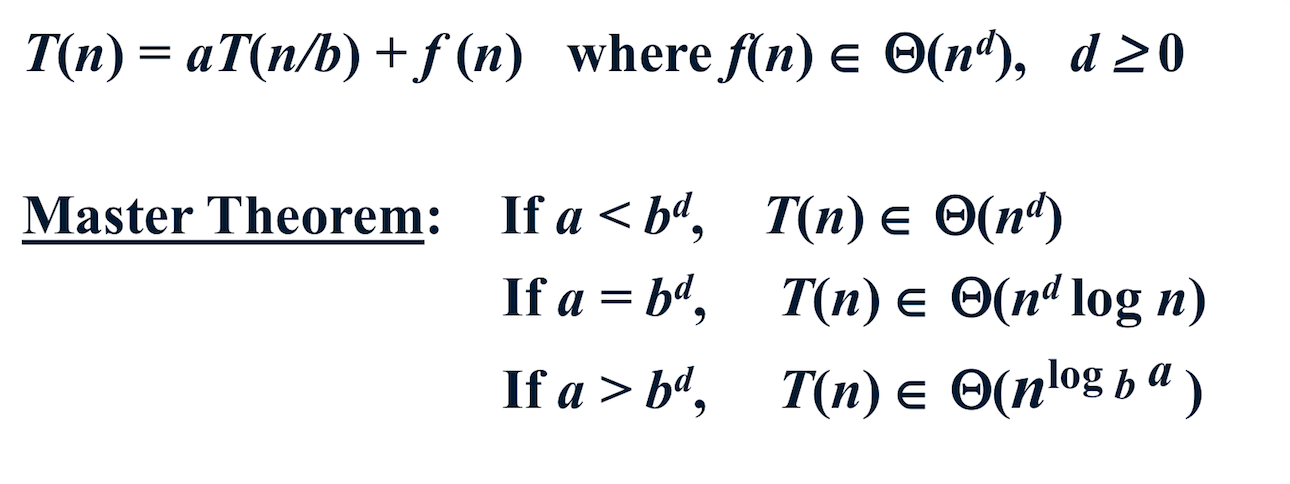例如
考虑归并排序
T(n) = 2T(n/2)+O（n）
其中a=2，b=2，d=1；
所以有：a=b^d即：
T(n)为O（nlogn）

### 补充方法

对于T(n) = aT(n/b) + f(n)
我们可以通过g(n)与f(n)比较来得到结果
其中g(n) = n^(loga(b))

若g(n)/f(n)>lg(n) -> T(n) = g(n)
若g(n)=f(n)       -> T(n) = lg(n)* f(n)或lg(n) *g(n)
若f(n)/g(n)>lg(n) -> T(n) = f(n)

注意对于该范围之外的master理论无法求解

展开全文递归 复杂度
• ## 解递归方程

千次阅读 2018-05-10 16:54:10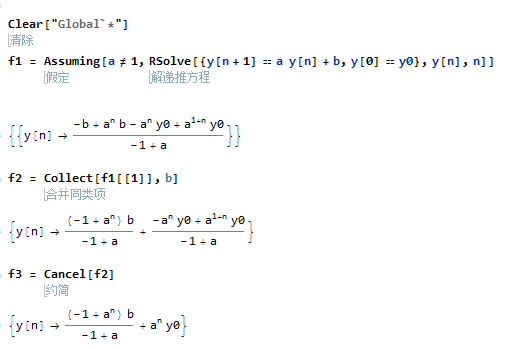展开全文• 算法复杂性经常描述为递归方程，解递归方程得到算法复杂性的具体表示 用特征方程解递归方程 用生成函数解递归方程 用递推方法解递归方程
• 递归方程 递归方程之前提到过，就是部分算法在求解的过程中使用了将一个问题划分成几个等价的小问题，在这个过程中，我们就可以列出一个等式。 （如归并排序中，将一个大数组拆分成两个小数组分别计算，然后用O(n)的...

## 递归方程

递归方程之前提到过，就是部分算法在求解的过程中使用了将一个问题划分成几个等价的小问题，在这个过程中，我们就可以列出一个等式。
（如归并排序中，将一个大数组拆分成两个小数组分别计算，然后用O(n)的代价合并，
T(n) = T(n/2)+O(n)就是一个递归方程）

那么，在得到一个递归方程之后，我们应该如何求解问题呢？

## 替换法/代入法

我们都知道，归并排序的时间复杂度为O(nlogn)，那么我们以后看到和上面给出的归并排序差不多的递归方程时，我们可能心中就有了一些猜想。
没错，这个方式就是通过经验经行猜测
比如T(n) = 2T(n/2 + 17) + n，因为我们之前说过时间复杂度这个东西和常数项等关系不大，所以我们自然会将其和nlog进行联系，那么此时我们就需要带入，进行验证。

需要注意，在使用数学归纳法的时候，我们是可以舍弃前几项的
因为算法的时间复杂度并非严格的数学计算，我们可以忽略很多东西，而且在θ等标记符的定义过程中我们也强调了n0的含义。

但是，猜也是需要积累经验的，对一些奇怪的问题我们可能很难做到一次猜对，很自然，我们可以考虑先猜一个上下阶，然后不断逼近的方式。
那么我们就需要一些常见的时间复杂度的大小比较：
O(1) < O(logn) < O(n) < O(nlogn) < O(n2) < O(n3）……随后是指数，阶乘函数是最大的。
需要注意的问题：

• 任意底数的logn都要比多项式低阶
• 不同底数的log是同阶的，只相差一个系数（换底公式）

随后是一个蛮奇怪的问题，就是在计算下面的时间复杂度时，我们的做法可能有一点不同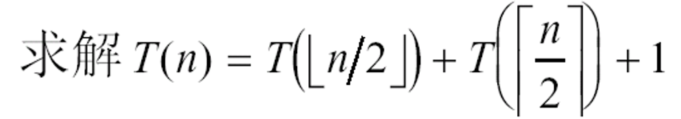我们可以通过猜测的方式，达到O(n)的程度，但是当将O(n)带入时，会发现：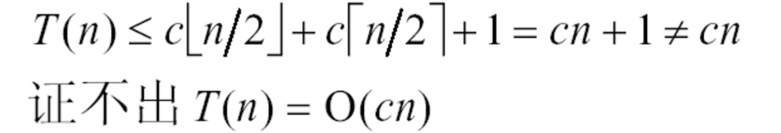此时我们最可能的想法就是要计算O(nlogn)了，因为低阶的已经不能证明了。
但正好相反，我们的做法是在原来的基础上减去一个常数项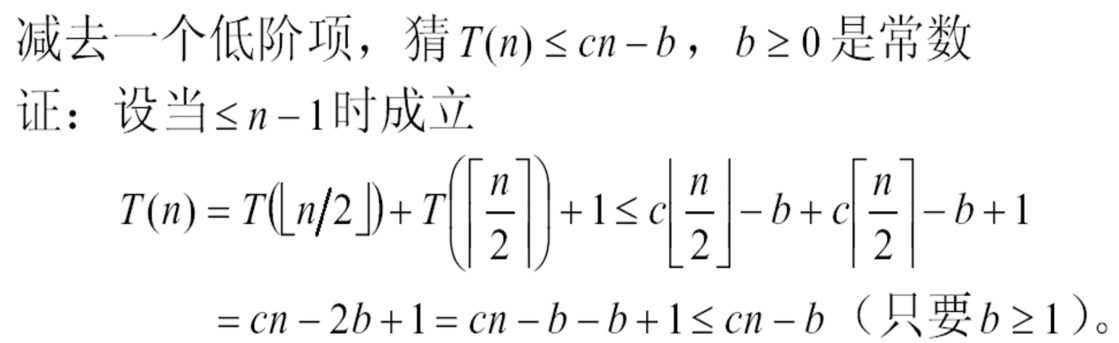这是因为等式距离cn只差了一个常数项，而递归方程的左边只有一个T函数，而右边则有两个，那么在用右边的式子代替左边时，我们就可以减去双倍的常数项，就可以保证等式成立了。

等等，还有一种比较常见的变型：
有时我们会遇见一些奇形怪状的问题，比如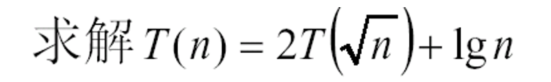那么此时就要想一下是否能通过变脸替换来解决问题了。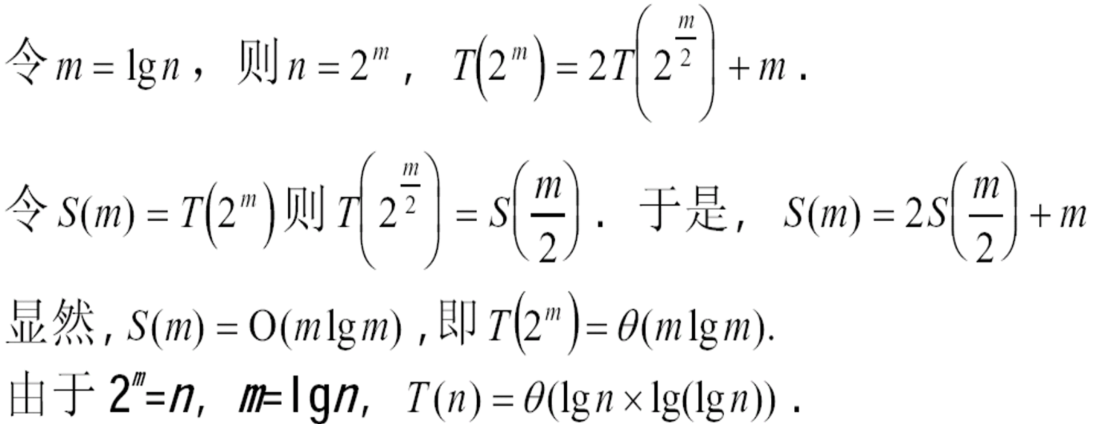## 递归树法

这个方法是非常有趣的，也是最接近所有方法的本质的。

T(n) = 3T(n/4)+θ(n2)

我们可以这样想象，首先我们有一个大小为n的原问题，然后我们将他拆分成三个n/4大小的子问题，然后用了θ(n2)的代价。
将θ(n2)写在树根，那么这个根节点就应该有3个孩子节点，每一个孩子节点的大小是n/4

当我们将这个过程继续下去，直到最后一层，每一个结点的大小都是T(1)时
（ T(1)的代价我们默认为O(1) ），我们就可以说这棵树到了叶子节点。
因为每一层我们都将原规模缩小为原来的1/4，然后每一层的个数都是原来的三倍，所以最后一层的叶子节点个数应为3log4n
使用换底公式，我们可以得到最后一层的代价为θ(nlog43）。（以4为底3的对数）

而每一次的拆分，我们都将代价写在非叶子结点上，那么将代价相加，
再加上最后的叶子节点代价，我们就可以得到整体的代价了。
（说白了就是我把所有代价都写在结点上了，叶子节点就是T(1) == θ(1)，你把所有的加起来就行了）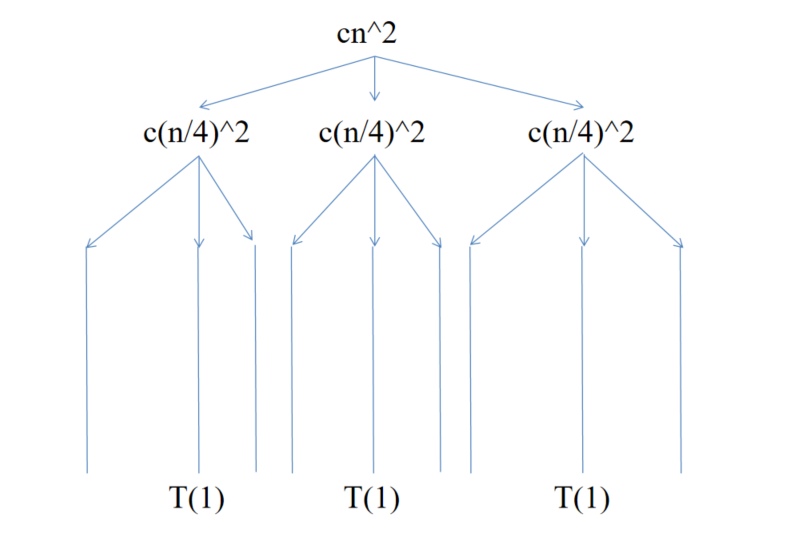（学校ppt上的照片有水印，自己做的将就一下吧T_T）

在得到了每一层的代价之后，求和就是高数的事了。

## 主方法（最简单的方式）

好叭，可能很多人都和我一样，高数早就还给了老师，所以大佬们就给出了一个十分简单的方式来计算这类的时间复杂度。
这里给出主方法的一个分析方式，但不属于证明！
在我们计算递归树的时候，如果真的动手去算了上面的代价，那么会发现非叶子节点是一个等比数列，而叶子节点是另外一种不同的计算方式。在之前我们提到过，时间复杂度只考虑最高阶，所以我们只需要比较两者阶的高低，就可以得到时间复杂度了。

主方法通式：T(n) = aT(n/b)+f(n)

非叶子节点：θ( f(n) ) 不管ab怎么样，最后都是f(n)带一串的常数
叶子节点 ：同上面的递归树，nlogba（以b为底a的对数）
也就是比较两者的大小了。
声明：这里面的大小需要满足多项式的大于，也就是n和nlogn这样主方法是不成立的

1. 当f(n) > nlogba 时，有T(n)=θ( f(n) )
2. 当f(n) < nlogba 时，有T(n)=θ( nlogba )
3. 当f(n) = nlogba 时，有T(n)=θ( f(n)*logn )
展开全文• ## 递归树求解递归方程

千次阅读 多人点赞 2021-07-31 09:56:08
首先了解一下这个递归式 T(n)=4T(n/2)+n 是什么意思： 4表示我们将一个问题分解为 4 个子问题 n/2表示每个子问题的规模是原问题的 1/2 n表示合并需要的额外计算时间 方法一可用主定理【Master定理】 主定理...
• ——求解形为T(n)=aT(n/b)+f(n)的递归方程 迭代法/Iteration方法 1.不断用递归方程的右部替换左部 2.每次替换，随着n的降低，在和式中多出一项 3.直到出现初值，停止迭代 4.将初值代入并对和式求和 5.可用数学...算法
• 目录如何求解T(N)=aT(N/b)+f(N)方法1：代入法(Substitution Method)方法2：递归树方法（Recursion Tree）方法3：主定理 (Master Theorem) 如何求解T(N)=aT(N/b)+f(N) 方法1：代入法(Substitution Method) 代入法求解...算法
• 大小为n的原问题分成若干个大小为n/b的子问题，其中a个子问题需要求解，而cnk是合并各个子问题的需要的工作量。 找出对应的abc，直接带入。 （1）直接转换 ...（3）求递归方程T(n)=4T(n/2)+n 的算法
• 主定理： 特殊递归方程 迭代展开 已知： T(n) = 2T(n-1)+1 T(1)=1 展开： T(n)=2T(n-1)+1=2(2T(n-2)+1)+1=2(2(2T(n-3)+1)+1)+1=...=== 等差数列之和： 等比数列之和： 调和数列之和： 故T(n)=O() ...算法
• 递归方程求解递归方程求解方法例子 递归方程求解 方法 例子
• 迭代法1. 直接迭代-Hanoi塔算法2. 换元迭代-二分归并排序3. 归纳验证 1. 直接迭代-Hanoi塔算法 ...将(关于k的函数)转换成关于 n 的函数 MergeSort(A,p,r) 输入∶数组 A【p…r】 输出∶按递增顺算法
• matlab利用递归求解差分方程时间：2018-5-23matlab利用递归求解差分方程function y = recur(a,b,n,x,x0,y0);%% y = recur(a,b,n,x,x0,y0)% solves for y[n] from:% y[n] + a1*y[n-1] + a2*y[n-2]...+ an*y[n-N]% = ...
• 递归方程的渐进阶的求法——套用公式法 这个方法为估计形如： T(n)=aT(n/b)+f(n) (6.17) 的递归方程解的渐近阶提供三个可套用的公式。(6.17)中的a≥1和b≥1是常数，f (n)是一个确定的正函数。 (6.17)是一类...
• 一、分而治之的思想 ... ③把这些小实例的组合成原始大实例的 二、实际应用之找出假币 问题描述 一个袋子有16个硬币，其中只有一个是假币，这个假币比其他的真币重量轻（其他所有真币的重量都是相同的）...分而治之
• ## 递归方程求解法

千次阅读 2019-07-03 12:13:21
递归方程求解法 特征方程求解 特征方程法本质上是先猜后证的方法。我们猜测方程的得形式为 xnx^nxn ，然后带入递归式，求解 xxx。 k阶齐次线性常系数 给出的条件有递归关系和初始： {f(n)=a1f(n−1)+a2f(n−2)+...
• T(n)=c1T(n-1)+c2T(n-2)+…+ ckT(n-k)+f(n)，n≥k (6.18)的递归方程。其中ci (i=l，2，…，k)为实常数，且ck≠0。它可改写为一个线性常系数k阶非齐次的差分方程：T(n)-c1T(n-1)- c2T(n-2)-…-ckT(n-k)=f(n)，n≥k ...
• - 60- 中国科技信息2006年第3期 CHINA SCIENCE AND TECHNOLOGY INFORMATION Feb.2006 科 技 论 坛 }} }} 引言 在计算机科学中，递归概念经常用于递归调用方面，即函数或过程自己调用自己。用递归调用的算法就是递归...
• 递归(recursion)算法的运行时间常用递归表达式...例一：T(n) = T(n-1)+1：T(n) =T(n-1)+1 = [T(n-2)+1]+1 = T(n-2)+2 =T(n-3)+3 = … = T(n-n)+n = n故 O(n) = n例二：T(n) = T(n-1)+n-1：T(n) =T(n-1)+n-1 = ...
• 解递归方程的方法 递归树（Recursion tree） 替代法（Substitution method） Master方法（Master method） 递归树 解T(n) = 2T(n/2) + cn, 其中 c > 0 为常数 递归方程中的问题 归并排序 分成两部分排序的时间...算法
• 7递归方程的渐进阶的求法——代入法[汇编].pdf
• ：替换法 到这里假设,则上式等于 2. T(n)=4T(n/2)+n :主方法，a=4,b=2,f(n)=n, 因此,可知,满足主方法第一条定理 所以 3. T(n)=4T(n/2)+c ：替换法 到这里假设，则上式等于,如果T(1)=1，则有 4. .....
• 转载于:https://www.cnblogs.com/xiu68/p/8168751.html
• 1） 求解上述特征方程的根，得到递归方程的通 2）利用递归方程初始条件，确定通中待定系数，得到递归方程 考虑2种情况： 1）特征方程的k个根不相同 2）特征方程有相重的根 特征方程的k个根不相同：假设...math
• ## 分治算法（递归）

千次阅读 2020-05-13 12:43:54
分治算法（递归）一、基本概念二、基本思想及策略三、分治法适用的情况四、分治法的基本步骤五、分治法的复杂性分析六、可使用分治法求解的一些经典问题七、依据分治法设计程序时的思维过程 一、基本概念 在计算机...
• ## 主方法求解递归式

千次阅读 多人点赞 2019-07-23 19:59:21
title: 主方法求解递归式 date: 2019-07-22 23:49:10 tags: 主方法求解递归式   在分析递归的算法时，主方法可以较快的计算出算法的时间复杂度 主方法可以用于满足以下形式的递归式。 T(n)=aT(n/b)+f(n)T(n)...时间复杂度 主方法 算法分析 算法导论
• T(1) = c 的递归关系， 有如下结论： if (a > b^k) T(n) = O(n^(logb(a))); if (a = b^k) T(n) = O(n^k*logn); if (a < b^k) T(n) = O(n^k); 对于：T(n) = 25T(n/5)+n^2 a=25 b = 5 k=2 有：a==b^k 故T(n)=O...算法 时间复杂度
• 分治策略递归式时间复杂度的求解方法主要有三种：代入法、递归树和主方法。其中主方法为求解递归式T(n)=aT(n/b)+f(n)T(n)=aT(n/b)+f(n)T(n)=aT(n/b)+f(n)提供了一种“菜谱”式的求解方法。 公式：T(n)=aT(n/b)+f(n)T...算法导论 分治策略 主方法 时间复杂度...